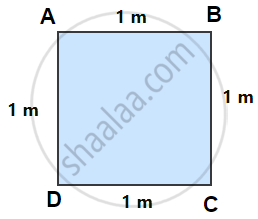# Perimeter of Squares

#### formula

Perimeter of a square = 4 × length of a side.

# Perimeter of a Square:Perimeter of the Square = Sum of the lengths of its four sides.

= AB + BC + CD + DA

= AB + AB + AB + AB....(All the four side of square are equal)

= 4 × AB

= 1m + 1 m + 1 m + 1 m = 4 × 1 m

= 4 m

Perimeter of the Square = Side + Side + Side + Side

i.e., Perimeter of a square = 4 × length of a side.

#### Example

Pinky runs around a square field of side 75 m, Bob runs around a rectangular field with length 160 m and breadth105 m. Who covers more distance and by how much?

Distance covered by Pinky in one round = Perimeter of the square
= 4 × length of a side
= 4 × 75 m
= 300 m

Distance covered by Bob in one round = Perimeter of the rectangle
= 2 × (length + breadth)
= 2 × (160 m + 105 m)
= 2 × 265 m
= 530 m

Difference in the distance covered = 530 m – 300 m = 230m.

Therefore, Bob covers more distance by 230 m.

#### Example

The perimeter of a rectangle of length 28 cm and breadth 20 cm is equal to the perimeter of a square. What is the length of the side of that square?

Perimeter of rectangle = 2 (length + breadth)
= 2 (28 + 20)
= 96

If the side of that square is a then 4a = 96

Perimeter of square = 96

4a = 96

∴ a = = 96/4 = 24.

Side of the square is 24 cm.

If you would like to contribute notes or other learning material, please submit them using the button below.